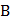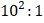# Consider the following two statementsandand identify the correct choice given in the answer (A) Duddells thermo-galvanometer is suitable to measure direct current only (B) Thermopile can measure temperature differences of the order ofa) Bothandare true b) Bothandare false c)is true butis false d)is false butis true

## Question ID - 151246 :- Consider the following two statementsandand identify the correct choice given in the answer (A) Duddells thermo-galvanometer is suitable to measure direct current only (B) Thermopile can measure temperature differences of the order ofa) Bothandare true b) Bothandare false c)is true butis false d)is false butis true

3537is false butis true

Next Question :
 The approximate ratio of resistances in the forward and reverse bias of the-junction diode is a)b)c)d)